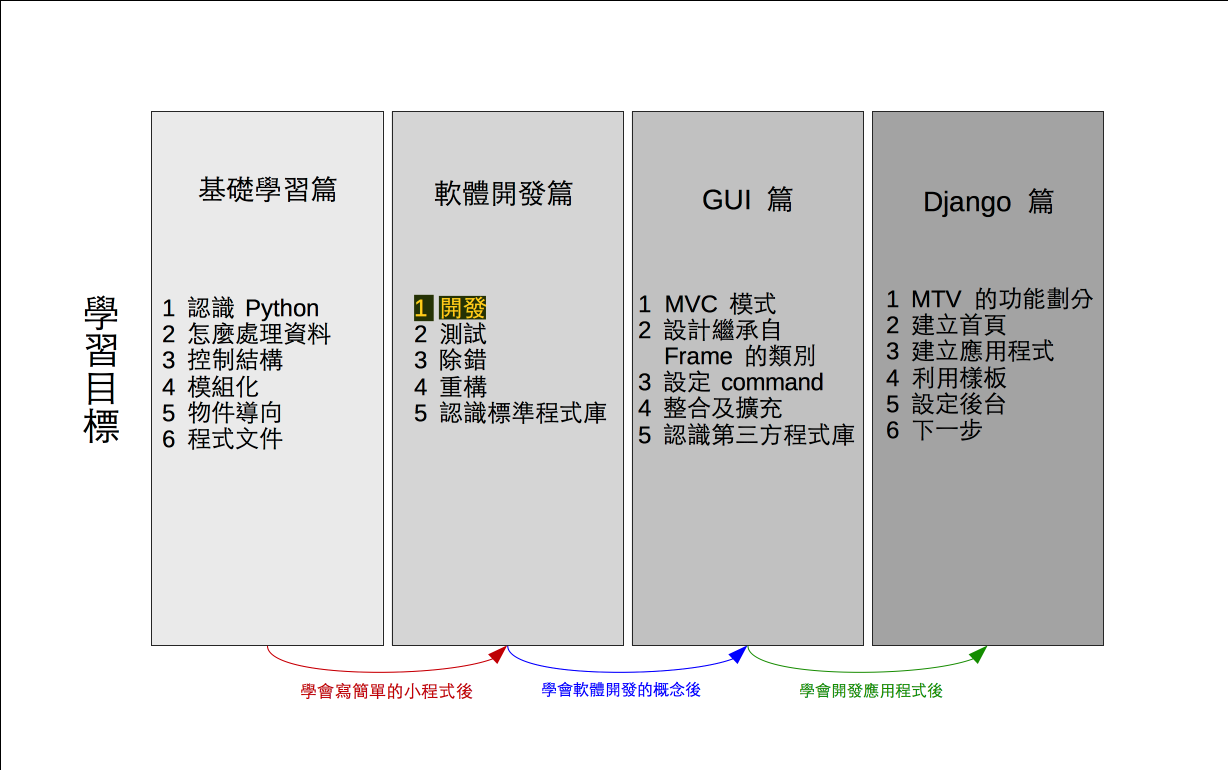# 單元 18 - 編碼

～～學習進度表～～There is no spoon.
×

 0 1 2 3 4 5 6 7 8 9 10 11 12 13 14 15 16 17 18 19 20 21 22 23 24 25 q z i r a j s b k t c l u d m v e n w f o x g p y h

``code = "qzirajsbktcludmvenwfoxgpyh"``

``"There is no spoon."``

``````# 使用 randint()
import random

# 定義 Encrypt 類別
class Encrypt:
def __init__(self):
self.setcode()

def setcode(self):
# 取得 a 、 b 值
a = 0
b = 0
while a % 2 == 0:
a = random.randint(0, 9)
b = random.randint(0, 9)

# 利用公式建立密碼表
self.code = ""
c = "a"
i = 0
while i < 26:
x = c
y = ord(x) * a + b
m = y % 26
self.code += chr(m + 97)
c = chr(ord(c) + 1)
i += 1

def getcode(self):
return self.code

# 編碼的方法
def toEncode(self, str):
# 暫存編碼結果的字串
result = ""

# 利用迴圈走完參數字串的所有字元
for c in str:
# 判斷該字元是否為英文小寫字母
# 若是英文小寫字母就進行編碼轉換
c1 = ord(c) >= 97
c2 = ord(c) <= 122
if c1 and c2:
m = ord(c) - 97
result += self.code[m]
else:
result += c

# 結束回傳編碼過的字串
return result

# 解碼的方法
def toDecode(self, str):
pass

# 測試部分
if __name__ == '__main__':
e = Encrypt()
print()
print(e.getcode())
s1 = "There is no spoon."
print("Input : " + s1)
s2 = e.toEncode(s1)
print("Encode: " + s2)
print()

#《程式語言教學誌》的範例程式
# http://kaiching.org/
# 檔名：encrypt06.py
# 功能：示範利用 Python 設計 Encrypt 類別
# 作者：張凱慶 */``````

toEncode() 接收一個字串 str參數 (parameter) ，也回傳一個新字串 resultstr 就是要編碼的字串，而 result 則是編碼過的字串

``````# 利用迴圈走完參數字串的所有字元
for c in str:
# 判斷該字元是否為英文小寫字母
# 若是英文小寫字母就進行編碼轉換
c1 = ord(c) >= 97
c2 = ord(c) <= 122
if c1 and c2:
m = ord(c) - 97
result += self.code[m]
else:
result += c``````

``````# 測試部分
if __name__ == '__main__':
e = Encrypt()
print()
print(e.getcode())
s1 = "There is no spoon."
print("Input : " + s1)
s2 = e.toEncode(s1)
print("Encode: " + s2)
print()``````

 \$ python encrypt06.py pyhqzirajsbktcludmvenwfoxg Input : There is no spoon. Encode: Tazmz jv cl vullc. \$

1. 轉換表格利用串列來儲存，每個元素都是長度為 1 的英文字母字串，可用索引值存取元素值。
2. 編碼時利用迴圈依序取得要編碼字串的所有字元，先判斷是否為英文小寫字母，如果是英文小寫字母就進行編碼，如果不是就直接把該字元附加到暫存變數的最後。

1. 要怎麼判斷一個字元是英文小寫字母或大寫字母？
2. 為什麼可以用對照表格的方式進行轉換？

1. 承接上一個單元的猜數字遊戲，將新程式寫在 exercise1801.py 中，在遊戲迴圈中利用內建函數 len() 檢查使用者輸入的長度，若是長度不等於 4 就印出提示訊息進行下一輪。
2. 承上題，將答案 answer 改成串列，並以 "0""9" 十個字串當元素，用標準模組庫中 randomshuffle() 攪亂順序後取得前四個元素當遊戲答案。